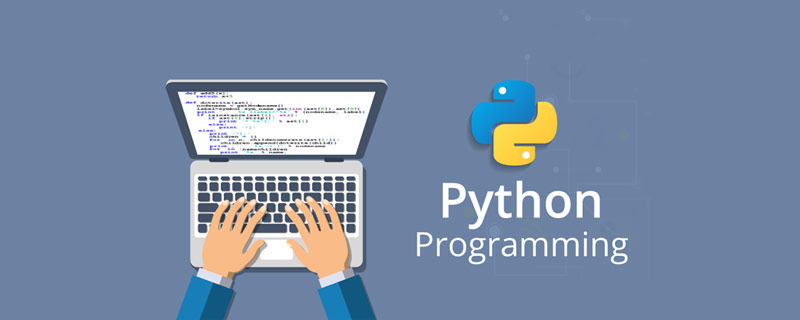# pandas妙招之 在DataFrame中通过索引高效获取数据_编程技术_亿码酷站

pandas数据处理专题的第三篇文章，我们一起来聊聊DataFrame中的索引。

## 数据对齐

`import numpy as npimport pandas as pddf1 = pd.DataFrame(np.arange(9).reshape((3, 3)), columns=list('abc'), index=['1', '2', '3'])df2 = pd.DataFrame(np.arange(12).reshape((4, 3)), columns=list('abd'), index=['2', '3', '4', '5'])复制代码`

## fill_value

DataFrame当中常用的运算符有这么几种：

fill_value这个参数在很多api当中都有出现，比如reindex等，用法都是一样的，我们在查阅api文档的时候可以注意一下。

## 空值api

### fillna

pandas除了可以drop含有空值的数据之外，当然也可以用来填充空值，事实上这也是最常用的方法。

fillna会返回一个新的DataFrame，其中所有的Nan值会被替换成我们指定的值。如果我们不希望它返回一个新的DataFrame，而是直接在原数据进行修改的话，我们可以使用inplace参数，表明这是一个inplace的操作，那么pandas将会在原DataFrame上进行修改。

`df3.fillna(3, inplace=True)复制代码`

## 总结

pandas妙招之 在DataFrame中通过索引高效获取数据

pandas妙招之 DataFrame基础运算以及空值填充

python基本运算符号有哪些

## python使用Matplotlib绘制饼图_亿码酷站_亿码酷站

python使用Matplotlib绘制饼图_亿码酷站_亿码酷站